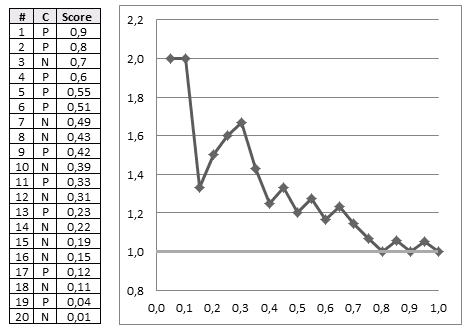# ML Wiki

## Cumulative Gain Chart

Gain Charts are used for Evaluation of Binary Classifiers

• also it can be used for comparing two or more binary classifiers
• the chart shows $\text{tpr}$ vs $\text{sup}$

### Motivating Example

Suppose we have a direct marketing campaign

• population is very big
• we want to select only a fraction of the population for marketing - those that are likely to respond
• we build a model that scores receivers - assigns probability that he will reply
• want to evaluate the performance of this model

### Cumulative Gain

Performance evaluation

• recall values that can be calculated for Evaluation of Binary Classifiers
• accuracy - but it's not enough here
• $\text{tpr}$ - True Positive Rate or Sensitivity
• $\text{tpr} = \cfrac{\text{TP}}{\text{TP} + \text{FN}}$
• fraction of examples correctly classified
• $\text{sup}$ - Support (Predictive Positive Rate)
• $\text{sup} = \cfrac{\text{TP} + \text{FP}}{N} = \cfrac{\text{predicted pos}}{\text{total}}$
• fraction of positively predicted examples

Suppose that we obtained the following data:

• Cls = actual class
• score = predicted score

Cls Score
N 0.01
P 0.51
N 0.49
P 0.55
P 0.42
N 0.7
P 0.23
N 0.39
P 0.04
N 0.19
P 0.12
N 0.15
N 0.43
P 0.33
N 0.22
N 0.11
N 0.31
P 0.8
P 0.9
P 0.6
$\Rightarrow$ sort $\Rightarrow$
# Cls Score
1 P 0.9
2 P 0.8
3 N 0.7
4 P 0.6
5 P 0.55
6 P 0.51
7 N 0.49
8 N 0.43
9 P 0.42
10 N 0.39
11 P 0.33
12 N 0.31
13 P 0.23
14 N 0.22
15 N 0.19
16 N 0.15
17 P 0.12
18 N 0.11
19 P 0.04
20 N 0.01
• sort the table by score desc
• max on top, min at bottom
• if model works well, expect
• responders at top
• non-responders at bottom
• the better the model
• the clearer the separation
• between positive and negative

Intuition

• suppose now we select top 20% records
• we see that out of 4 examples 3 of them are positive
• in total, there are 10 responders (positive classes)
• so with only 20% (4 records) we can target 3/10 = 30% responders
• we also can use a random model
• if you randomly sample 20% of records, you can expect to target only 20% your responders
• 20% of 10 = 2
• so we're doing better than random
• can do it for all possible fractions of our data set and get this chart:Best classifier

• the optimal classifier will score positives and negatives s.t. there's a clear separation between them
• in such a case the gain chart will always go up until it reaches 1, and then go left
•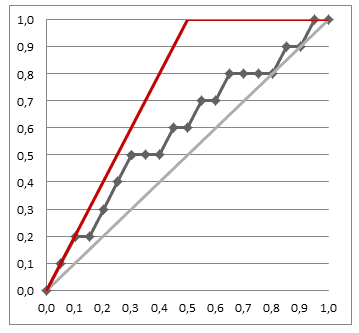• the closer our chart to the best one, the better our classifier is

### Gain Chart

So a gain chart shows

• Predicted Positive Rate (or support of the classifier)
• vs True Positive Rate (or sensitivity of the classifier)
•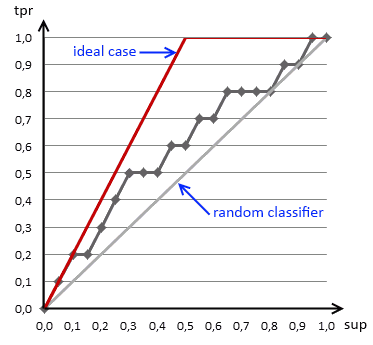• it says how much population we should sample to get the desired sensitivity of our classifier
• i.e. if we want to direct 40% of potential repliers to our targeting campaign, we should select 20%
• when we divide our data into two subsets, we can plot the charts for both
•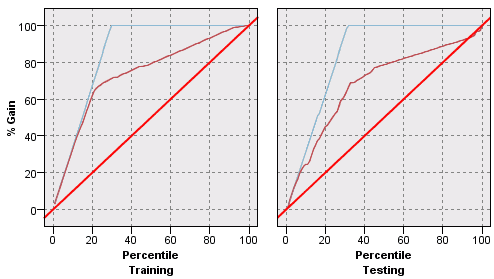• we can easily see if a classifier overfits on the test set, but underperforms on the testing

### Examples

Given

• 20 training examples, 12 negative and 8 positive
# Cls Score
1 N 0.18
2 N 0.24
3 N 0.32
4 N 0.33
5 N 0.4
6 N 0.53
7 N 0.58
8 N 0.59
9 N 0.6
10 N 0.7
11 N 0.75
12 N 0.85
13 P 0.52
14 P 0.72
15 P 0.73
16 P 0.79
17 P 0.82
18 P 0.88
19 P 0.9
20 P 0.92
$\Rightarrow$ sort(score)
# Cls Score
20 P 0.92
19 P 0.9
18 P 0.88
12 N 0.85
17 P 0.82
16 P 0.79
11 N 0.75
15 P 0.73
14 P 0.72
10 N 0.7
9 N 0.6
8 N 0.59
7 N 0.58
6 N 0.53
13 P 0.52
5 N 0.4
4 N 0.33
3 N 0.32
2 N 0.24
1 N 0.18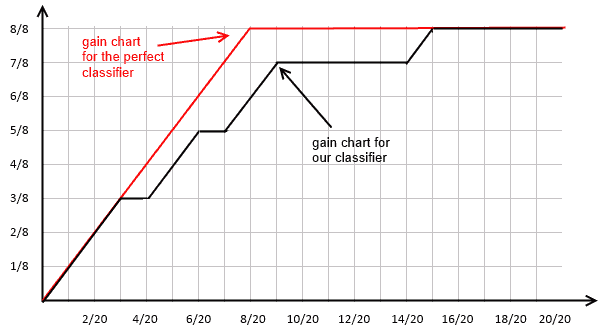### Comparing Binary Classifiers

Can draw two or more gain charts over the same plot

• and thus be able to compare two or more classifiers
•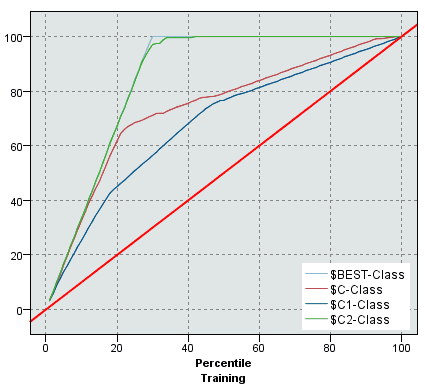• we see that one of the classifiers most likely overfits the training data
•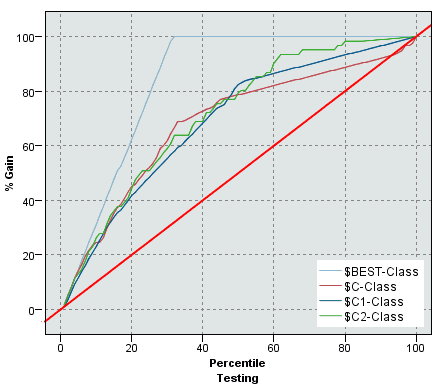• but when we test, we see that it performs as good (bas) as other classfiers

### Plotting Gain Chart in R

In R there's a package called ROCR  (for drawing ROC Curves)

install.packages('ROCR')
require('ROCR')


It can be used for drawing gain charts as well:

cls = c('P', 'P', 'N', 'P', 'P', 'P', 'N', 'N', 'P', 'N', 'P',
'N', 'P', 'N', 'N', 'N', 'P', 'N', 'P', 'N')
score = c(0.9, 0.8, 0.7, 0.6, 0.55, 0.51, 0.49, 0.43,
0.42, 0.39, 0.33, 0.31, 0.23, 0.22, 0.19,
0.15, 0.12, 0.11, 0.04, 0.01)

pred = prediction(score, cls)
gain = performance(pred, "tpr", "rpp")

plot(gain, col="orange", lwd=2)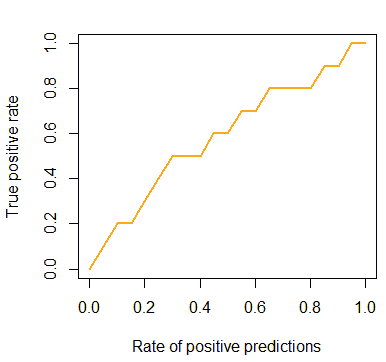But we also can add the baseline and the ideal line:

plot(x=c(0, 1), y=c(0, 1), type="l", col="red", lwd=2,
ylab="True Positive Rate",
xlab="Rate of Positive Predictions")
lines(x=c(0, 0.5, 1), y=c(0, 1, 1), col="darkgreen", lwd=2)

gain.x = unlist(slot(gain, 'x.values'))
gain.y = unlist(slot(gain, 'y.values'))

lines(x=gain.x, y=gain.y, col="orange", lwd=2)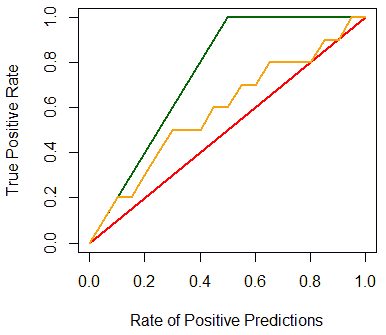## Cumulative Lift Chart

Lift charts show basically the same information as Gain charts

• $\text{ppr}$ Predicted Positive Rate (or support of the classifier)
• vs $\cfrac{\text{tpr}}{\text{ppr}}$ True Positive over Predicted Positive
•